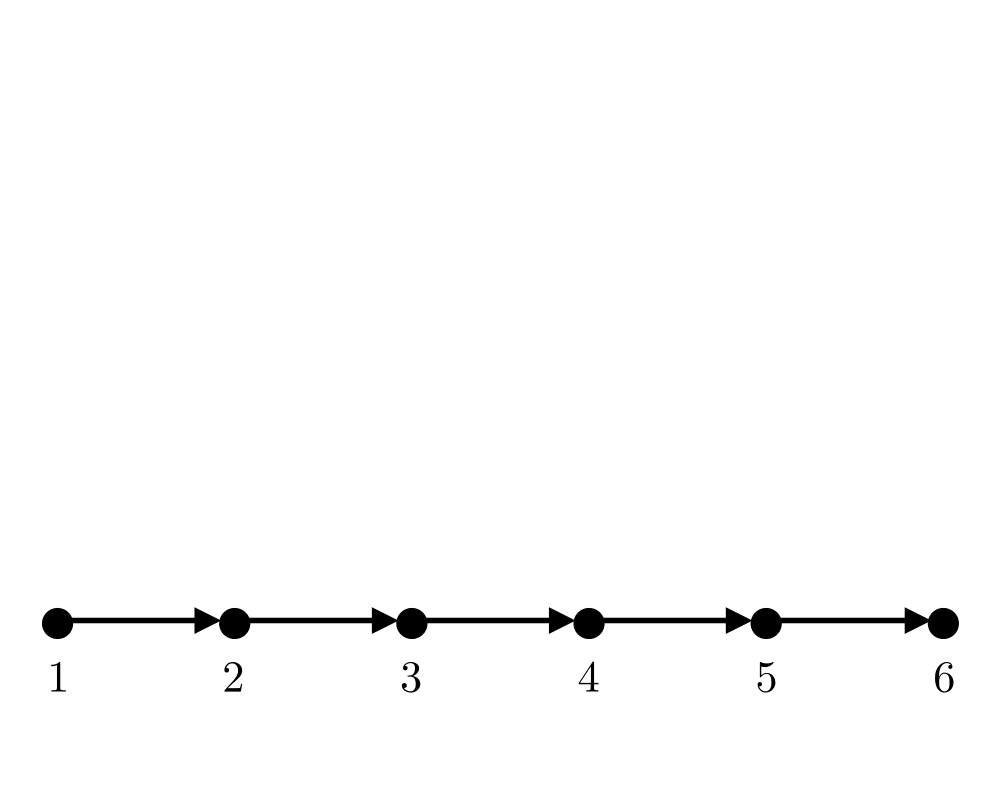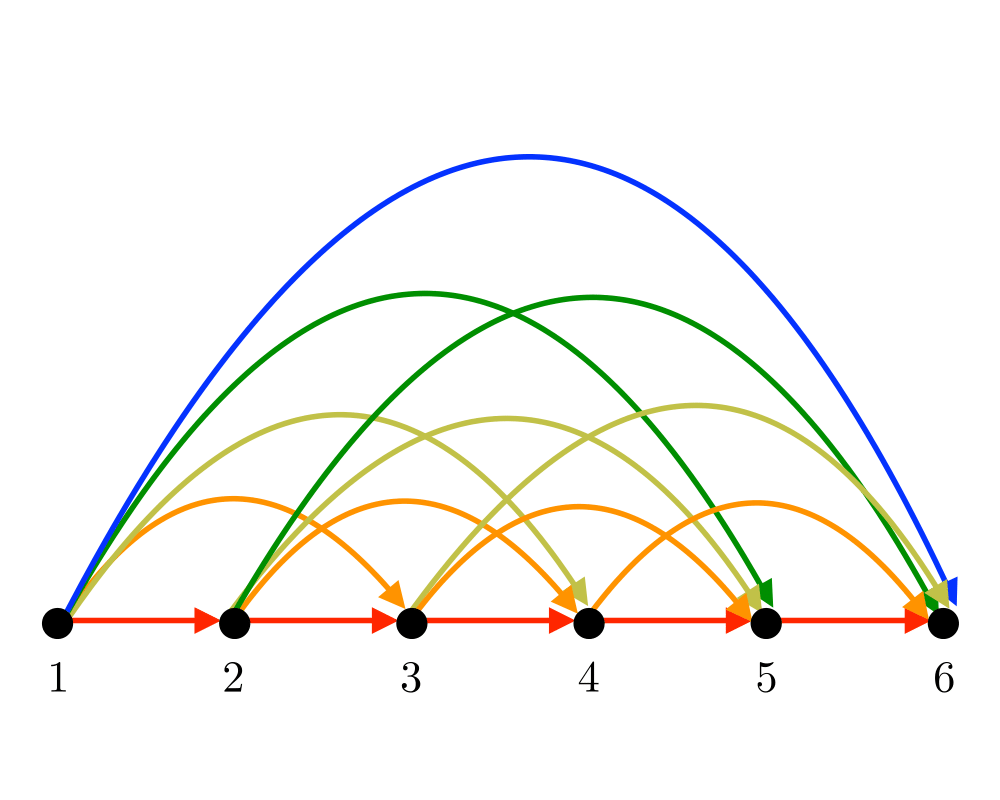# Transitive Closure and ReductionInputOutput

Input Description: A directed graph $$G=(V,E)$$.
Problem: For transitive closure, construct a graph $$G'=(V,E')$$ with edge $$(i,j) \in E'$$ iff there is a directed path from $$i$$ to $$j$$ in $$G$$. For transitive reduction, construct a small graph $$G'=(V,E')$$ with a directed path from $$i$$ to $$j$$ in $$G'$$ iff $$(i,j) \in E$$.

Excerpt from The Algorithm Design Manual: Transitive closure can be thought of as establishing a data structure that makes it possible to solve reachability questions (can I get to $$x$$ from $$y$$?) efficiently. After the preprocessing of constructing the transitive closure, all reachability queries can be answered in constant time by simply reporting a matrix entry. Transitive closure is fundamental in propagating the consequences of modified attributes of a graph $$G$$. For example, consider the graph underlying any spreadsheet model, where the vertices are cells and there is an edge from cell $$i$$ to cell $$j$$ if the result of cell $$j$$ depends on cell $$i$$. When the value of a given cell is modified, the values of all reachable cells must also be updated. The identity of these cells is revealed by the transitive closure of $$G$$. Many database problems reduce to computing transitive closures, for analogous reasons.

Go To Main Page